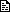## 优化递归的效率

1.尾递归转换成迭代

PHP代码
1. <?php
2. function  f( \$n = 0)
3. {
4.     if(\$n<=0)return 1;
5.     return \$n*f(\$n-1);
6. }
7. ?>

PHP代码
1. <?php
2. function f(\$n = 0)
3. {
4.     \$r=0;
5.     while\$n-- > 0)
6.         \$r *= \$n;
7.     return \$r;
8. }
9. ?>

2.动态规划

PHP代码
1. <?php
2. function Fib( \$n)
3. {
4.     if(\$n==1)return 1;
5.     if(\$n==0)return 0;
6.     return Fib(\$n-1)+Fib(\$n-2);
7. }
8.
9. //这个函数我没有测试。。。 我是指转换过来后
10. function Fib( \$n )
11. {
12.     \$f=1;\$f=0;
13.     for(\$i=0;\$i<=\$n;\$i++);
14.         f[\$i]=f[\$i-1]+f[\$i-2];
15.     \$r\$f[\$n];
16.     return \$r;
17. }
18.
19. ?>

a(n)=a(n-2)+a(n-4);

3.备忘录

4.内联

PHP代码
1. <?php
2. function Fib( \$n)
3. {
4.     if(\$n==1)return 1;
5.     if(\$n==0)return 0;
6.
7.     \$fn1 = \$fn2 = 0;
8.     if\$n-1==1)\$fn1=1;
9.     else if(\$n-1==0)\$fn1=0;
10.     else \$fn1=Fib(\$n-2)+Fib(\$n-3);
11.
12.     if(\$n-2==1)\$fn2=1;
13.     else if(\$n-2==0)\$fn2=0;
14.     else \$fn2=Fib(\$n-3)+Fib(\$n-4);
15.
16.     return \$fn1+\$fn2
17. }
18.
19. ?>

5.解递归

（１）解齐次递归方程F(0)=0和F(1)=1可以得出方程组（２）母函数Tags: php, 递归, 效率, 优化

« 上一篇 | 下一篇 »

## 只显示10条记录相关文章

PHP导入导出Excel方法 (浏览: 38479, 评论: 3)

PHP的XSS攻击过滤函数 (浏览: 35039, 评论: 2)
PHP中Eval的作用 (浏览: 34510, 评论: 4)
PHP常见错误（二） (浏览: 32910, 评论: 1)
PHP sendmail (浏览: 32266, 评论: 7)

## 1条记录访客评论

Post by peter on 2012, June 1, 6:25 PM#1